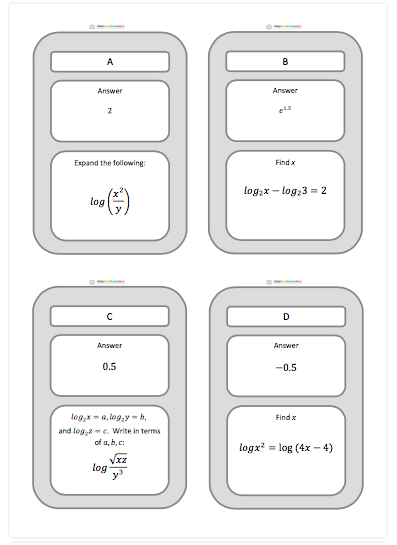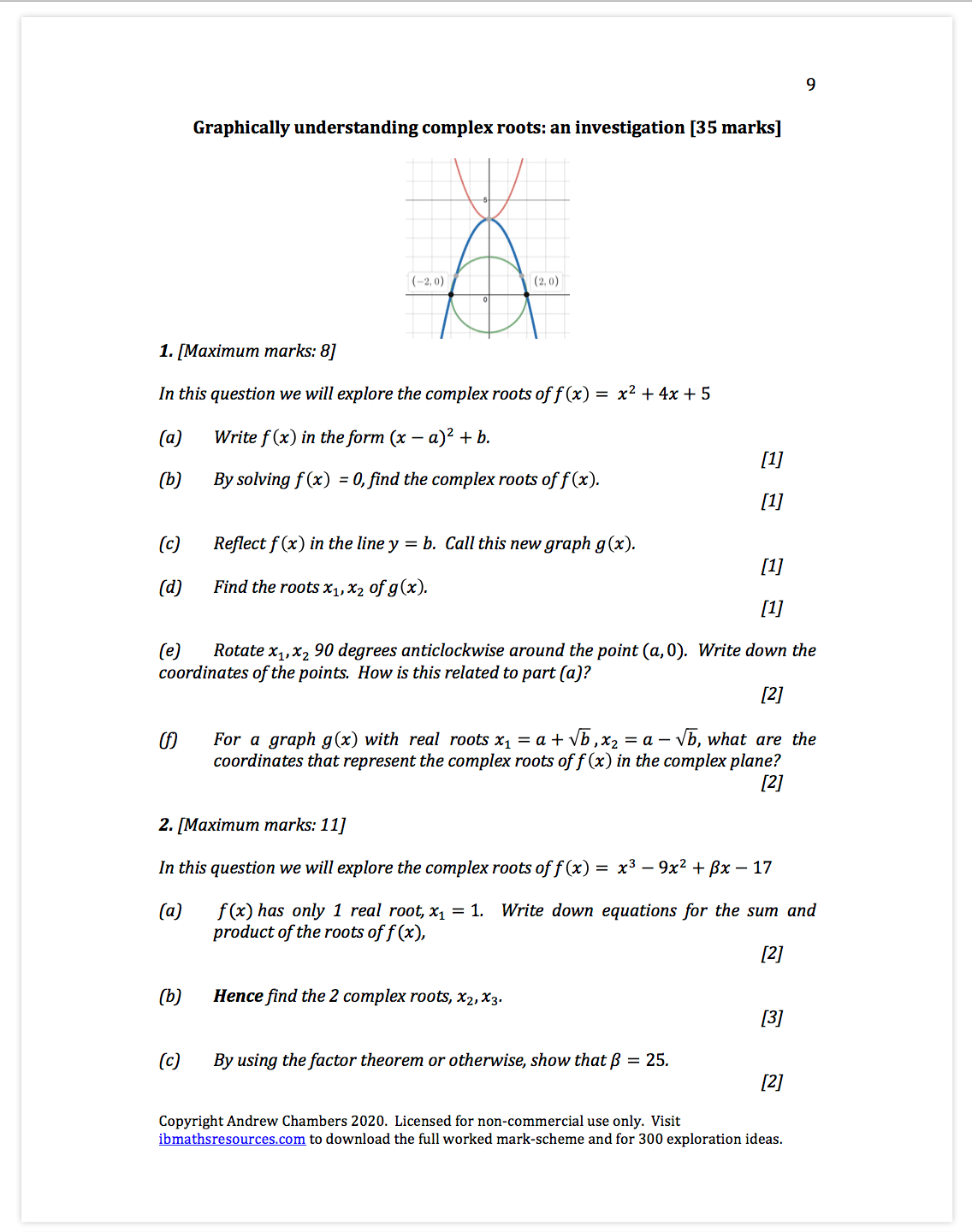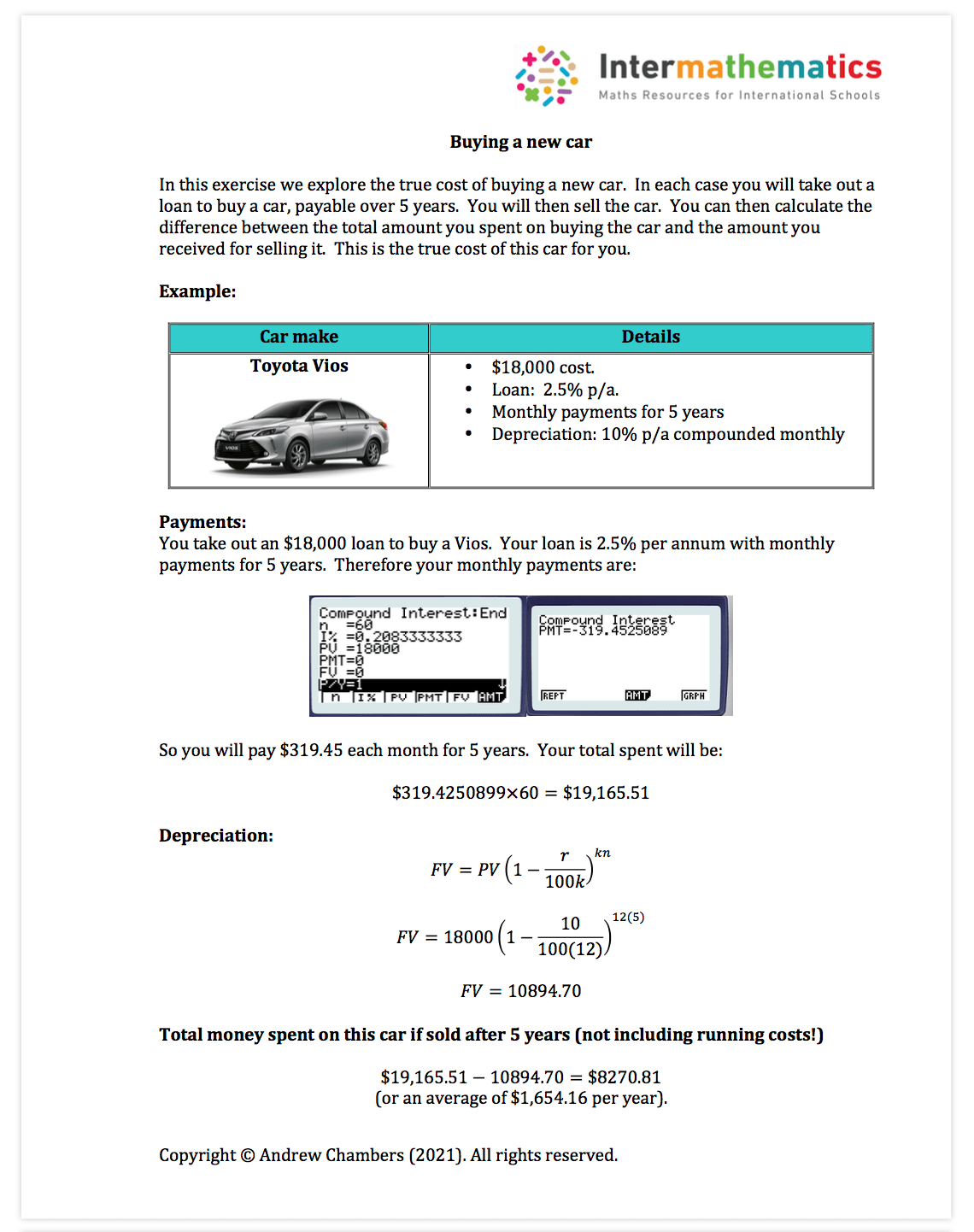# Algebra Free Content

Algebra content: Sequences, Binomials, Counting Principles, Proof, Exponents, Logs and Complex Numbers

Worksheets in the premium set: 45 worksheets (around 90 pdf pages of questions and around 200 pdf pages of fully typed solutions)

The algebra content contains a mixture of shorter answer questions and longer past paper style questions.

Sequences worksheet example:Sequences investigation example:Logs activity example (treasure hunt):Permutations and combinations example:Complex numbers example (Paper 3 investigation)Complex numbers example: classroom investigation and exploration ideaFinancial mathematics example: